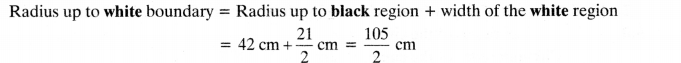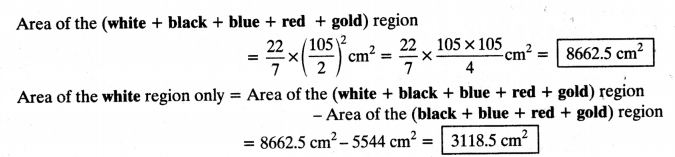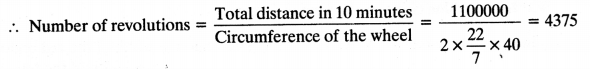NCERT Solutions for Class 10 Maths Chapter 12 Areas Related to Circles Ex 12.1 are part of NCERT Solutions for Class 10 Maths. Here we have given NCERT Solutions for Class 10 Maths Chapter 12 Areas Related to Circles Ex 12.1.

 Board CBSE Textbook NCERT Class Class 10 Subject Maths Chapter Chapter 12 Chapter Name Areas Related to Circles Exercise Ex 12.1 Number of Questions Solved 5 Category NCERT Solutions

## NCERT Solutions for Class 10 Maths Chapter 12 Areas Related to Circles Ex 12.1

Ex 12.1 Class 10 Question 1.
The radii of two circles are 19 cm and 9 cm respectively. Find the radius of the circle which has circumference equal to the sum of the circumferences of the two circles.
Solution:
Given: radius of 1st circle (R1) = 19 cm
∴ Circumference of 1st circle = 2πR1 = 2π(19) cm
Radius of 2nd circle (R2) = 9 cm
∴ Circumference of 2nd circle = 2πR2 = 2π(9) cm
Let radius of 3rd circle be R3
Circumference of 3rd circle = 2πR3
According to question,
2πR1 + 2πR2 = 2πR3
⇒ 2π(R1 + R2) = 2πR3
⇒ R1 + R2 = R3
⇒ 19 + 9 = R3
⇒ R3 = 28 cm

Class 10 Maths Chapter 12.1 Question 2.
The radii of two circles are 8 cm and 6 cm respectively. Find the radius of the circle having area equal to the sum of the areas of the two circles.
Solution:
Given: radius of 1st circle (R1) = 8 cm
Area of 1st circle = πR12 = π(8)2cm2
Radius of 2nd circle (R2) = 6 cm
Area of 2nd circle = πR22 = π(6)2 cm2
Let radius of 3rd circle be R3
Area of 3rd circle = πR32
According to question,
πR,2 + πR22 – πR32
⇒ R12 + R22 = R32  ⇒ (8)2 + i6)2 – R32
⇒ 64 + 36 = R32 ⇒ R3=  $$\sqrt{100}$$ = 10 cm

Areas Related To Circles Exercise 12.1 Question 3.
The figure depicts an archery target marked with its five scoring regions from the centre outwards as Gold, Red, Blue,
Black and White. The diameter of the region representing Gold score is 21 cm and each of the other bands is 10.5 cm wide. Find the area of each of the five scoring regions.Solution:
Diameter of the region representing gold score is 21 cm
⇒ Radius of the region representing gold region = $$\frac { 21 }{ 2 }$$ cmExercise 12.1 Class 10 Question 4.
The wheels of a car are of diameter 80 cm each. How many complete revolutions does each wheel make in 10 minutes when the car is travelling at a speed of 66 km per hour?
Solution:
Given: diameter of the wheels of the car = 80 cm
⇒ Radius of the wheel of the car = $$\frac { 80 }{ 2 }$$ = 40 cm
Circumference of the wheel = 2πr = 2 x $$\frac { 22 }{ 2 }$$ x 40 cm
Speed of the car = 66 km/h
Distance covered in 10 minutes =$$\frac { 66 x 10 }{ 60 }$$ = 11 km
= 11 x 1000 x 100 cm = 11,00,000 cmEx 12.1 Class 10 Maths Solution Question 5.
Tick the correct answer in the following and justify your choice: If the perimeter and the area of a circle are numerically equal, then the radius of the circle is
(a) 2 units
(b) n units
(c) 4 units
(d) 7 units
Solution:
Let radius of the circle = r units
Perimeter of the circle = 2πr
Area of the circle = πr2
According to question,
Perimeter of the circle = Area of the circle
⇒ 2πr = πr2
⇒ r = 2 units
Hence, option (a) is correct.

We hope the NCERT Solutions for Class 10 Maths Chapter 12 Areas Related to Circles Ex 12.1 help you. If you have any query regarding NCERT Solutions for Class 10 Maths Chapter 12 Areas Related to Circles Ex 12.1, drop a comment below and we will get back to you at the earliest.